# Kummer surface

In algebraic geometry, Kummer's quartic surface is an irreducible algebraic surface over a field K of characteristic different then 2, which is a hypersurface of degree 4 in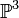$\mathbb{P}^3$ with 16 singularities; the maximal possible number of singularities of a quartic surface. It is a remarkable fact that any such surface is the Kummer variety of the Jacobian of a smooth hyperelliptic curve of genus 2; i.e. a quotient of the Jacobian by the Kummer involution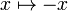$x\mapsto-x$. The Kummer involution has 16 fixed points: the 16 2-torsion point of the Jacobian, and they are the 16 singular points of the quartic surface.

## Geometry of the Kummer surface

### Singular quartic surfaces and the double plane model

Let$K\subset\mathbb{P}^3$ be a quartic surface, and let p be a singular point of this surface. Identifying the lines in$\mathbb{P}^3$ thorugh the point p with$\mathbb{P}^2$, we get a double cover from the blow up of K at p to$\mathbb{P}^2$; this double cover is given by sending$q\neq p\mapsto\overline{pq}$, and any line in the tangent cone of p in K to itself. The ramification locus of the double cover is a plane curve C of degree 6, and all the nodes of K which are not p map to nodes of C.

By the genus degree formula, the maximal number possible number of nodes on a sextic curve is obtained when the curve is a a union of 6 lines, in which case we have 15 nodes. Hence the maximal number of nodes on a quartic is 16, and in this case they are all simple nodes (to show that p is simple project from another node). A quartic which obtains these 16 nodes is called a Kummer Quartic, and we will concentrate on them below.

Since p is a simple node, the tangent cone to this point is mapped to a conic under the double cover. This conic is in fact tangent to the six lines (w.o proof). Conversely, given a configuration of a conic and six lines which tangent to it in the plane, we may define the double cover of the plane ramified over the union of these 6 lines. This double cover may be mapped to <mathbb>\mathbb{P}^3[/itex], under a map which blows down the doubel cover of the special conic, and is an isomorphism elsewhere (w.o. proof).

### The double plane and Kummer varieties of Jacobians

Starting from a smooth curve C of genus 2, we may identify the Jacobian Jac(C) with Pic2(C) under the map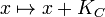$x\mapsto x+K_C$. We now observe two facts: Since C is a hyperelliptic curve the map from the symmetric product Sym2C to Pic2C, defined by$\{p,q\}\mapsto p+q$, is the blow down of the diagonal to the canonical divisor class. Moreover, the canonical map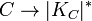$C\to|K_C|^*$ is a double cover. Hence we get a double cover$Kum(C)\to Sym^2|K_C|^*$.

This double cover is the one which already appeared above: The 6 lines are the images of the odd symmetric theta divisors on Jac(C), while the conic is the image of the blown-up 0. The conic is isomorphic to the canonical system via the isomorphism$T_0 Jac(C)\cong |K_C|^*$, and each of the six lines is naturally isomorphic to the dual canonical system | KC | * via the identification of theta divisors and translates of the curve C. There is a 1-1 correspondence between pairs of odd symmetric theta divisors and 2-torsion points on the Jacobian given by the fact that$(\Theta+w_1)\cap(\Theta+w_2)=\{w_1-w_2,0\}$, where w1,w2 are Weierstrass points (which are the odd theta characteristics in this in genus 2). Hence the branch points of the canonical map$C\mapsto |K_C|^*$ appear on each of these copies of the canonical system as the intersection points of the lines and the tangency points of the lines and the conic.

Finally, since we know that every Kummer quartic is a Kummer variety of a Jacobian of a hyperelliptic curve, we show how to reconstruct Kummer quartic surface directly from the Jacobian of a genus 2 curve: The Jacobian of C maps to the complete linear system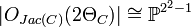$|O_{Jac(C)}(2\Theta_C)|\cong\mathbb{P}^{2^2-1}$ (see the article on Abelian varieties). This maps factors through the Kummer variety as a degree 4 map which has 16 nodes at the images of the 2-torsion points on Jac(C).

## Level 2 structure

### Kummer's 166 configuration

There are several crucial points which relate the geometric, algebraic, and combinatorial aspects of the configuration of the nodes of the kummer quartic:

• Any symmetric odd theta divisor on Jac(C) is given by the set points$\{q-w|q\in C\}$, where w is a Weierstrass point on C. This theta divisor contains six 2-torsion points: w' − w such that w' is a Weierstrass point.
• Two odd theta divisors given by Weierstrass points w,w' intersect at 0 and at ww'.
• The translation of the Jacobian by a two torsion point is an isomorphism of the Jacobian as an algebraic surface, which maps the set of 2-torsion points to itself.
• In the complete linear system | 2ΘC | on Jac(C), any odd theta divisor is mapped to a conic, which is the intersection of the Kummer quartic with a plane. Moreover, this complete linear system is invariant under shifts by 2-torsion points.

Hence we have a configuration of 16 conics in$\mathbb{P}^3$; where each contains 6 nodes, and such that the intersection of each two is along 2 nodes. This configuration is called the 166 configuration.

### The Weil Pairing

The 2-torsion points on an Abelian variety admit a symplectic bilinear form called the Weil pairing. In the case of Jacobians of curves of genus two, every non trivial 2-torsion point is uniquely expressed as a difference between two of the six Weierstrass points of the curve. The Weil pairing is given in this case by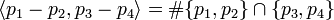$\langle p_1-p_2,p_3-p_4\rangle=\#\{p_1,p_2\}\cap\{p_3,p_4\}$. One can recover a lot of the group theoretic invariants of the group SP4(2) via the geometry of the 166 configuration.

### Group theory, algebra and geometry

Below we give a list of group theoretic invariants and their geometric incarnation in the 166 configuration.

• Polar lines
• Apolar complexes
• Klien's 6015 configuration